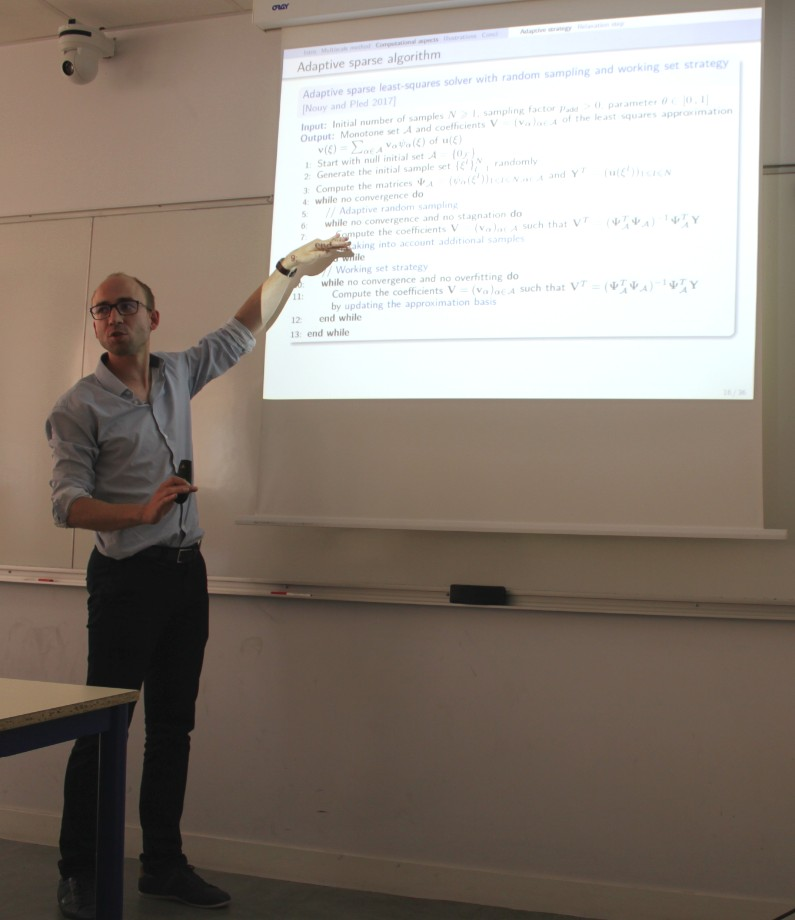A multiscale method with patches for the propagation of localized uncertainties in (semi-) linear elliptic and parabolic equations

Au Cnam, Paris, le 30 juin 2017 à 14h

Florent Pled
Maître de Conférences, Laboratoire de Modélisation et Simulation Multi-Échelle (MSME),
Université Paris-Est Marne-la-Vallée (UPEM), FranceUncertainty quantification in structural computation has gained an ever increasing interest in the scientific community during the last decades. The propagation of localized uncertainties through stochastic computational models is critical for the design and analysis of mechanical structures and components. The input uncertainties in the computational model may stem from intrinsic variabilities in the material properties or epistemic variabilities resulting from partial or limited information about the geometry, the boundary or initial conditions. Classical monoscale approaches require either local refinement or enrichment techniques to cope with the high complexity of multiscale stochastic problems. On the contrary, multiscale coupling approaches based on substructuring, domain decomposition or multigrid methods have been designed to solve such intractable high-dimensional problems.

A multiscale method based on patches has been recently proposed in  for the solution of linear stochastic multiscale models (with localized uncertainties) and extended to a broader class of non-linear stochastic multiscale models (with localized uncertainties and non-linearities) in . It relies on a partition of the domain into several subdomains of interest (called patches) containing the different sources of uncertainties and possible non-linearities, and a complementary subdomain. The multiscale solution is then computed using a global-local iterative algorithm that involves the solution of a sequence of linear global problems (with possibly deterministic operators and uncertain right-hand sides) over a deterministic domain and (non-)linear local problems (with uncertain operators and right-hand sides) over patches.

In the present work, the method is extended to time evolution problems featuring localized uncertainties. The convergence of the iterative algorithm is analyzed in the stationary case. The proposed multiscale approach offers the possibility to use different global and local computational models with suitable space and time discretizations (finite element spaces, numerical time integration schemes) as well as stand-alone global and local solvers. At the local level, the stochastic problems are solved using sampling-based approaches combined with adaptive sparse approximation methods  in order to efficiently derive sparse representations of high-dimensional stochastic local solutions with controlled accuracy. The performances of the multiscale domain decomposition method are illustrated through numerical experiments carried out on a stationary non-linear diffusion-reaction stochastic problem and a transient linear advection diffusion-reaction stochastic problem with localized random material heterogeneities.

Références

  M. Chevreuil, A. Nouy, E. Safatly, A multiscale method with patch for the solution of stochastic partial differential equations with localized uncertainties, Computer Methods in Applied Mechanics and Engineering, 255, 255–274, 2013. doi  A. Nouy, F. Pled, A multiscale method for semi-linear elliptic equations with localized uncertainties and non-linearities, ESAIM: Mathematical Modelling and Numerical Analysis (M2AN), submitted, 2017.  A. Chkifa, A. Cohen, C. Schwab, Breaking the curse of dimensionality in sparse polynomial approximation of parametric PDEs, Journal de Mathématiques Pures et Appliquées, 103 (2), 400–428, 2015. doi

Laboratoire de Mécanique des Structures et des Systèmes Couplés - LMSSC